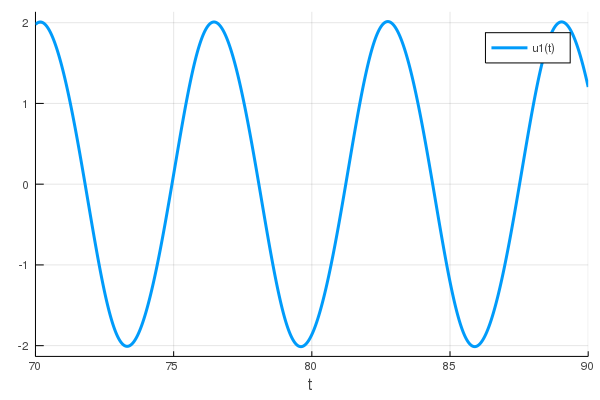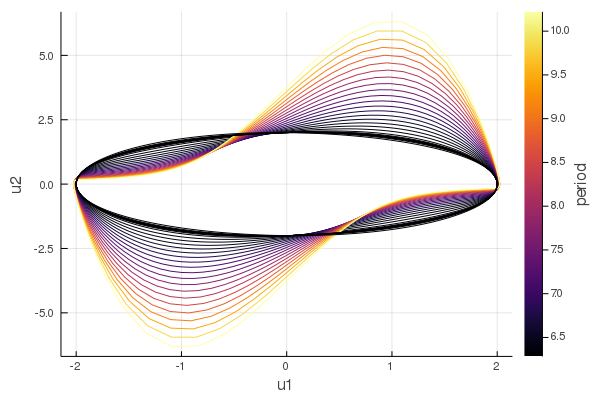Continuation of limit cycles of the van der Pol oscillator

# Continuation of limit cycles of the van der Pol oscillator

``````using Bifurcations
using Bifurcations: LimitCycleProblem
using Bifurcations.Examples.DuffingVanDerPol

using Plots
using OrdinaryDiffEq: Tsit5, remake``````

Create an `ODEProblem` and solve it:

``````ode = remake(
DuffingVanDerPol.ode,
p = DuffingVanDerPol.DuffingVanDerPolParam(
d = 0.1,
),
u0 = [1.0, 1.8],
tspan = (0.0, 90),
)
sol = solve(ode, Tsit5())

plt_ode = plot(sol, vars=1, tspan=(70, 90))``````Let's find a point (approximately) on the limit cycle and its period:

``````using Roots: find_zero
t0 = find_zero((t) -> sol(t) - 1, (80, 83))
t1 = find_zero((t) -> sol(t) - 1, (t0 + 3, t0 + 7))
x0 = sol(t0)
@assert all(isapprox.(x0, sol(t1); rtol=1e-2))
x0``````
``````2-element Array{Float64,1}:
1.000000000000025
1.822900836879164``````

Then a `LimitCycleProblem` can be constructed from the `ode`.

``````num_mesh = 50
degree = 5
t_domain = (0.01, 4.0)  # so that it works with this `num_mesh` / `degree`
prob = LimitCycleProblem(
ode, DuffingVanDerPol.param_axis, t_domain,
num_mesh, degree;
x0 = x0,
l0 = t1 - t0,
de_args = [Tsit5()],
)``````

As the limit cycle is only approximately specified, solver option `start_from_nearest_root = true` must be passed to start continuation:

``````solver = init(
prob;
start_from_nearest_root = true,
max_branches = 0,
)
@time solve!(solver)``````
``````BifurcationSolver <Continuous>
# sweeps             : 2
# points             : 35
# branches           : 1
# saddle_node        : 1
``````

By default, `plot_state_space` plots limit cycles colored by its period:

``plt_lc = plot_state_space(solver)``